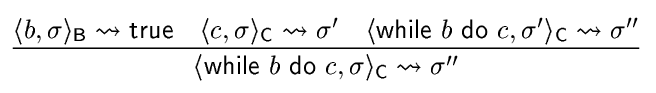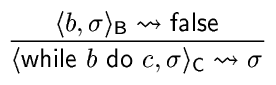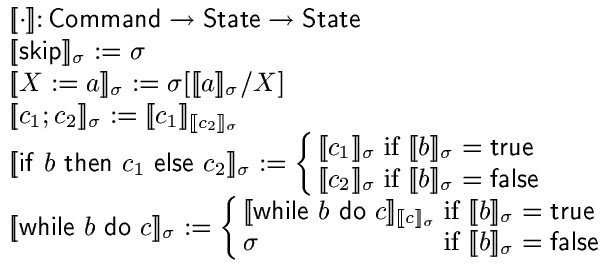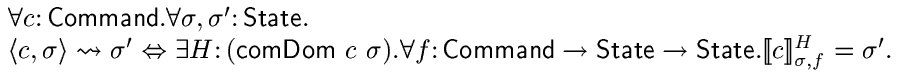# Highlights from the Workshop on Termination and Type TheoryTuesday Seminar at OGI on 12 December, 2002.

# Workshop on Termination and Type Theory

• It was held at the Hjortviken conference hotel, near Göteborg, on 14-15 November 2002.• It attracted more people than expected. For example, Peter Aczel and Per Martin-Löf were there.
• It was a rather informal workshop, without proceedings. The program, and abstracts of some of the talks are available from the workshop's web page.

## A session is about to start# Some interesting talks

I pick three talks that I liked and talk about them.

# Conor McBride: Seeing and Doing

### or Termination for Free

A common programming style:
• Pick a simple data structure.
• Describe your algorithm by saying first do this, then do that.
A better style:
• Understand the structure of your computation and design your data structures to match.
• This allows you to see the structure of the computation, since it follows the data structure.

# Conor McBride: Seeing and Doing

• In the former approach (doing), you typically need general recursion, and termination has to be proved "after the fact".
• In the latter (seeing), structural recursion is enough! You get a termination proof for free!
• The more powerful the type system, the better your data structure can can capture the structure of the computations. Dependent types are powerful.
Next: examples in Haskell.
Seeing and Doing :

# Example: sorting by structural recursion

Sorting is easy to define with structural recursion:

insert :: Ord a => a -> [a] -> [a] sort :: Ord a => [a] -> [a] insert x [] = [x] insert x (y:ys) = if x<=y then x:y:ys else y:insert x ys sort [] = [] sort (x:xs) = insert x (sort xs)

However, there are more efficient sorting algorithms...

Seeing and Doing : Example :

# Sorting efficiently

Resorting to general recursion, we can define a better sorting function:

quicksort :: Ord a => [a] -> [a] quicksort [] = [] quicksort (x:xs) = quicksort (filter (<x) xs)++ x: quicksort (filter (>=x) xs)

But this is almost structurally recursive. We only need to get rid of the call to `filter` in the recursive call to `quicksort`.

Seeing and Doing : Example :

# Sorting efficiently with structural recursion

We combine `quicksort` and `filter` into one function:

qsf p xs = quicksort (filter p xs)
By simple transformations, we obtain:
qsf p [] = [] qsf p (x:xs) = if p x then qsf (\x'->p x' && x'<x) xs++ x: qsf (\x'->p x' && x'>=x) xs else qsf p xs

We used the law: `filter p1 . filter p2 === filter (\x->p2 x && p1 x)`

Seeing and Doing : Example :

# Sorting efficiently with structural recursion

• In this case, it is fairly easy transform the generally recursive function into a structurally recursive function.
• Next: an example of a more generally applicable approach.
Seeing and Doing :

# From doing to seeing

• How does the computation proceed? What is the structure?
• For quicksort, in what state is the machine that executes quicksort after each step? `quicksort [3,5,2,4]`
• 3 : ⊥
• 3 : 5 : ⊥
• ...
Seeing and Doing :

# From doing to seeing

The structure that emerges is a binary search tree. This reveals the following alternative formulation of `quicksort`:

treesort xs = flatten (foldr insert Empty xs) data Tree a = Empty | Node a (Tree a) (Tree a) insert a Empty = Node a Empty Empty insert a (Node b l r) = if a<=b then Node b (insert a l) r else Node b l (insert a r) flatten t = flatten' t [] where flatten' Empty = id flatten' (Node a l r) = flatten' l . (a:) . flatten' r

# Ana Bove: Modelling General Recursion in Type Theory

• Based on a practical approach to handling general recursion in type theory.
• Defines a small functional language (called FP), with general recursion.
• Defines a mechanical translation from FP to type theory.
• The method allows for general recursion, including mutual recursion and nested recursion.
• The translated functions look very similar to the original functions.
• Termination proofs are kept apart from the function definitions. This means that
• You can prove things about functions without first having to prove that they terminate.
• Partial functions can be handled.
• Non-trivial example: unification.
Modelling General Recursion in Type Theory :

# General recursion in type theory

## The old way

• Any total, generally recursive function can be represented in type theory by using the accessibility predicate, provided you have a size measure that decreases in the recursive calls. [Acz77,Nor88]
• The method can be cumbersome, since termination proofs becomes intertwined with the function definition.
Modelling General Recursion in Type Theory :

# General recursion in type theory

## The new way

• Each generally recursive function is translated into two parts:
1. A special purpose accessibility predicate, or domain predicate. It is inductively defined, based on the structure of the original function.
2. A translated function that instead of general recursion on the original domain uses only structural recursion over the proof that the argument satisfies the domain predicate.
Modelling General Recursion in Type Theory :

# An example

• The original function:
quicksort :: Ord a => [a] -> [a] quicksort [] = [] quicksort (x:xs) = quicksort (filter (<x) xs)++ x: quicksort (filter (>=x) xs)
• The domain predicate:
Dqs :: [a] -> Prop QsNil :: Dqs [] QsCons :: (x::a)->(xs::[a])-> Dqs (filter (<x) xs)-> Dqs (filter (>=x) xs) -> Dqs (x:xs)
Modelling General Recursion in Type Theory :

# An example

• The original function:
quicksort :: Ord a => [a] -> [a] quicksort [] = [] quicksort (x:xs) = quicksort (filter (<x) xs)++ x: quicksort (filter (>=x) xs)
• The translated function
quicksort :: Ord a => (xs::[a]) -> Dqs xs -> [a] quicksort [] QsNil = [] quicksort (x:xs) (QsCons x xs p q) = quicksort (filter (<x) xs) p ++ x: quicksort (filter (>=x) xs) q
Modelling General Recursion in Type Theory :

# An example

Assuming you have a termination proof,
qsAll :: (xs::[a]) -> Dqs xs
you can define
qs :: Ord a => [a] -> [a] qs xs = quicksort xs (qsAll xs)
with the same type as the original function.
Modelling General Recursion in Type Theory :

# What about mutual recursion?

• Mutually recursive functions give rise to
1. simultaneously defined inductive domain predicates,
2. mutually recursive translated functions.
• No problem!
Modelling General Recursion in Type Theory :

# What about nested recursion

• Consider the following:
nest :: N -> N nest O = O nest (S n) = nest (nest n)
• The mechanically constructed domain predicate becomes
Dnest :: N -> Prop NestO :: Dnest O NestS :: (n::N) -> Dnest n -> Dnest (nest n) -> Dnest (S n)
• The domain predicate needs to refer to the function! The above definition is not correct!
Modelling General Recursion in Type Theory :

# What about nested recursion

• We need simultaneous inductive-recursive definitions! [Dyb00]
• We get:
NestO :: Dnest O NestS :: (n::N) -> (p::Dnest n) -> Dnest (nest n p) -> Dnest (S n) nest :: (n::N) -> Dnest n -> N nest O NestO = O nest (S n) (NestS n p q) = nest (nest n p) q
• No problem!
• But Coq does not support this kind of definitions.
General Recursion in Type Theory :

# A non-trivial example: Unification

## Part 1: data types

type Var = Int type Fun = Int data Term = Var Var | Fun Fun [Term] type Assignment = (Var,Term) type Substitution = [Assignment] type Equation = (Term,Term) type Equations = [Equation]
General Recursion in Type Theory :

# Unification

## Part 2: the unification function

unify :: Equations -> Maybe Substitution unify eqs = unify' eqs [] unify' :: Equations -> Substitution -> Maybe Substitution unify' [] s = Just s unify' ((Var x,Var y):eqs) s | x==y = unify' eqs s unify' ((Var x,t):eqs) s | x `elem` vars t = Nothing | not (x `elem` vars t) = unify' (substEqs x t eqs) ((x,t):substS x t s) unify' ((Fun f ts,Var x):eqs) s = unify' ((Var x,Fun f ts):eqs) s unify' ((Fun f ts1,Fun g ts2):eqs) s | f/=g || length ts1/=length ts2 = Nothing | f==g && length ts1==length ts2 = unify' (zip ts1 ts2++eqs) s
Is unify' structurally recursive?
General Recursion in Type Theory : Unification :

## Termination?

• Fortunately, there is a measure that allows us to prove that unify' terminates for all arguments.
• ...
General Recursion in Type Theory : Unification :

## Domain predicateGeneral Recursion in Type Theory : Unification :

## Proofs

Ana's thesis contains formal proofs of the following:
• unify is total, .e.g., all lists satisfy UniAcc.
• unify returns `Nothing` only if the equations aren't unifiable.
• The domain of the resulting substitution is a subset of the variables that occur in the equations.
• The resulting substitution is idempotent.
• The resulting substitution is a most general unifier.

# Yves Bertot: Type-theoretic functional semantics

• Defines a little imperative language IMP (from [Winskel 1993]).
• Gives an operational semantics, represented as an inductively defined relation in Coq.
• Gives a denotational semantics, represented as functions in Coq. Resolves problems with general recursion and nontermination.
• Formally proves that the operational semantics is equivalent to the functional semantics.
• Extracts an interpreter (as OCaml code) from the denotational semantics.
Type-theoretic functional semantics :

# The language IMP

Arithmetic expressions:

a ::= n | X | a₀ + a₁ | a₀ - a₁ | a₀ * a₁

Boolean expressions:

b ::= true | false | a₀ = a₁ | a₀a₁ | ¬b | b₀b₁ | b₀b₁

Commands:

c ::= skip | X := a | c₀ ; c₁ | if b then c₀ else c₁ | while b do c

Type-theoretic functional semantics :

# Operational Semantics

No surprises here.
• For arithmetic expressions: relates an expression and a state to a number.
• For boolean expressions: relates an expression and a state to a boolean.
• For commands: relates a command and a state to the new state.
• Examples:Type-theoretic functional semantics :

# Denotational Semantics

• For arithmetic expressions: Expr -> State -> Nat
• For boolean expressions: BExpr -> State -> Bool
• For commands: Command -> State -> State
But there is a problem with a naive definition of the denotation function for commands...
Type-theoretic functional semantics : Denotational Semantics :

# Denotation of Commands

## Naive attemptType-theoretic functional semantics : Denotational Semantics :

# Denotation of Commands

## Solution

Untie the recursive knot!Type-theoretic functional semantics :

# Relating the two semantics• Fk is just `iter F k`, where `iter` is iterated function composition, defined by structural recursion on k.
• Usually fix-point iteration starts from ⊥. Here it is instead shown that you can start from an arbitrary function g.
Type-theoretic functional semantics :

# Relating the two semantics

• Proof of the implication in the opposite direction requires the formulation of a domain predicate, `comDom`, for the denotation function.
• An extra complication arises because of nested recursion and the absence of Dybjer's simultaneous inductive-recursive definitions in Coq.
• The equivalence of the operational and the denotational semantics is now stated as follows:Type-theoretic functional semantics :

# Program extraction

Extraction of function F is simple, since it doesn't contain any proof components. The interpreter is then obtained by
interp :: Command -> State -> State interp = fix F

# Conclusions

• It could be that the most groundbreaking talk was one of the talks I didn't understand...

[|]